## 9.4.10 Arrays

Mathematical structures such as matrices and vectors require elements to be arranged in rows and columns. Just as we can align material in rows and columns in text mode using the tabular environment (§4.6. Aligning Material in Rows and Columns), we can do the same in maths mode using the array environment. The array environment has the same format as the tabular environment, however it must be in maths mode. The column half-gaps are given by the length register \arraycolsep (analogous to \tabcolsep).

Example:

$\begin{array}{rrr} 0 & 1 & 19\\ -6 & 10 & 200 \end{array}$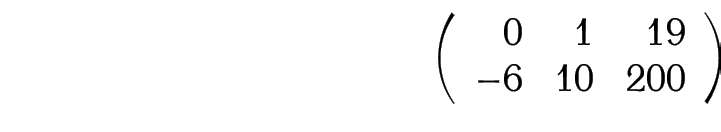A vertical rule can be added using | in the column specifier. For example:Example (Cases):

This example uses an invisible delimiter:

$f(x) = \left\{ \begin{array}{rl} -1 & x < 0\\ 0 & x = 0\\ +1 & x > 0 \end{array} \right.$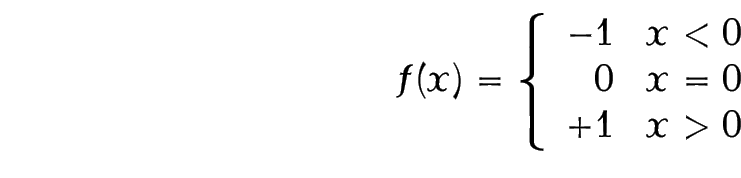This can be rewritten more compactly using the amsmath cases environment:

$f(x) = \begin{cases} -1 & x < 0\\ 0 & x = 0\\ +1 & x > 0 \end{cases}$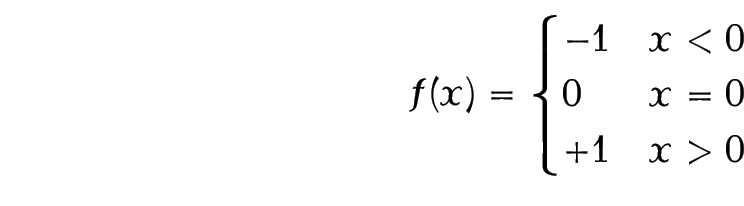The amsmath package provides some convenient environments to typeset matrices: pmatrix, bmatrix, Bmatrix, vmatrix and Vmatrix. These are similar to the array environment except there is no argument, and they add (respectively)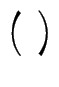,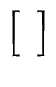,,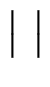anddelimiters. There is also the matrix environment that doesn't have any delimiters.

Example:The amsmath package also provides the environment smallmatrix designed for in-line use. You need to add any delimiters explicitly.

Example:

Here is a small matrix
\begin{math}
\left(
\begin{smallmatrix}
a & b\\
c & d
\end{smallmatrix}
\right)
\end{math}
in a line of text.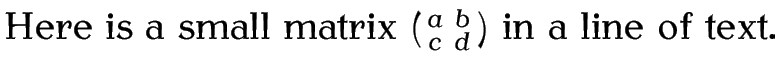This book is also available as A4 PDF or 12.8cm x 9.6cm PDF or paperback (ISBN 978-1-909440-00-5).

© 2012 Dickimaw Books. "Dickimaw", "Dickimaw Books" and the Dickimaw parrot logo are trademarks. The Dickimaw parrot was painted by Magdalene Pritchett.